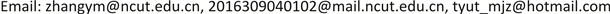1北方工业大学计算机学院，北京

2北京华龙通科技有限公司，北京1. 引言

2. 基于改进运动模板和直方图匹配的视频典型目标检测方法

2.1. 传统运动模板法

(1) 扫描mhi图像直到寻找到当前时间滞留范围内的像素点，该像素点为最新当前时间的轮廓区域的边界点；

(2) 继续搜索当前时间最新轮廓区域的边界以寻找紧邻当前边界外围的最近运动区域。当发现这样的运动区域时，采用逐级洪水泛滥法分割感兴趣目标的当前位置，逐级洪水泛滥分割算法步骤如下：

① 选取mhi图像中的一点标记作为种子像素点；

② 检测种子像素点的颜色，并对该像素点进行8方向搜索，检测其邻近像素点的颜色是否满足设置的阈值范围，若满足，则将该像素点的颜色填充为种子像素点的颜色值，即合并当前像素点与种子像素点构成连通区域，否则不进行处理；

③ 重复①、②直至mhi图像都被处理；

④ 采用阈值分割法从mhi图像中分割感兴趣的目标，本文统计图像中各像素点的值，并计算图像中各像素点值的均值与方差，将均值与3倍方差之和作为分割阈值；

(3) 保存(2)分割的感兴趣目标的当前位置；

(4) 重复步骤(2)和(3)，直至找到所有区域。

2.2. 直方图匹配法

HSV颜色空间将颜色分为3个独立的空间向量，H表示色彩的基本属性；S表示色彩的纯度，S值越高其色彩越纯；V表示色彩的明亮程度。由于色彩的明亮程度易受光照的影响，在有光照影响的条件下，V的检测值会与真实值相差很大，因此，本文选择使用H、S色彩向量作为直方图匹配算法的基础色彩向量。

H = ( H 1 , H 2 , ⋯ , H 10 ) (2)

S = ( S 1 , S 2 , ⋯ , S 5 ) (3)

H、S色彩直方图中每个柱的值可以表示为 H i ( i = 1 , 2 , ⋯ , k ) ， S j ( j = 1 , 2 , ⋯ , m ) ，每个柱值的计算方法如公式(4)、(5)所示。

H i = ∑ f i ( h ( x , y ) ) , i = 1 , 2 , ⋯ , k (4)

S j = ∑ f i ( h ( x , y ) ) , j = 1 , 2 , ⋯ , m (5)

D B ( p , q ) = − ln ( B C ( p , q ) ) (6)

B C ( p , q ) = ∑ x ∈ X p ( x ) q ( x ) (7)

2.3. 融合改进运动模板和直方图匹配的视频典型目标检测方法

P k ( x , y ) = | f k ( x , y ) − f k − 1 ( x , y ) | (8)

R k ( x , y ) = { 0 , P k ( x , y ) < T 3 1 , P ( x , y ) ≥ T 3 (9)

R k ( x , y ) = { 1 , R k ( x , y ) ∩ R k + 1 ( x , y ) = 1 0 , otherwise (10)

1) 设定初始阈值 T h 0 、迭代数 T = 0 及每步阈值增加的步长step，阈值的设定如公式(11)所示；

T h 0 = ( median [ P k ( x , y ) ] + max [ P k ( x , y ) ] + min [ P k ( x , y ) ] ) / 4 (11)

2) 按照公式(12)计算分割后两类间的差异度；

S = ( T h 0 − μ 1 ) ( μ 2 − T h 0 ) ( μ 2 − μ ) 2 (12)

3) 检查是否达到迭代数，若达到迭代数则停止迭代，并选取最大的 s 对应的阈值 T h 0 作为分割阈值 ，否则继续执行步骤(4)；

4) 按照公式(13)增加阈值，公式(14)增加迭代数，并重复步骤(2)、(3)；

T h 0 = T h 0 + step (13)

T = T + 1 (14)

{ D B = D B H * w 1 + D B S * w 2 w 1 + w 2 = 1 (15)

3. 实验结果与分析

A ( x ) = { A 1 i + 1 | χ − μ | < α λ A 2 i + 1 | χ − μ ′ | < α λ (16)

{ μ = { μ Α 1 i > Α 2 i μ ′ Α 1 i ≤ Α 2 i ω κ = ω Μ (17)

{ μ Κ = 0 σ Κ 2 = σ Μ ω Κ = ε (18)

{ ω k , t = ( 1 − α ) ω k , t − 1 + α μ k , t = ( 1 − ρ ) μ k , t − 1 + ρ χ t σ k , t = ( 1 − ρ ) σ k , t − 1 2 + ρ ( χ t − μ k , t ) (19)

The comparison result of three method

4. 结论